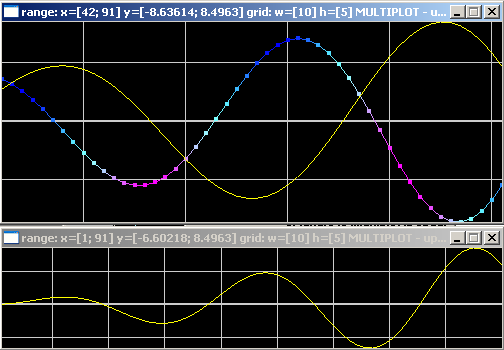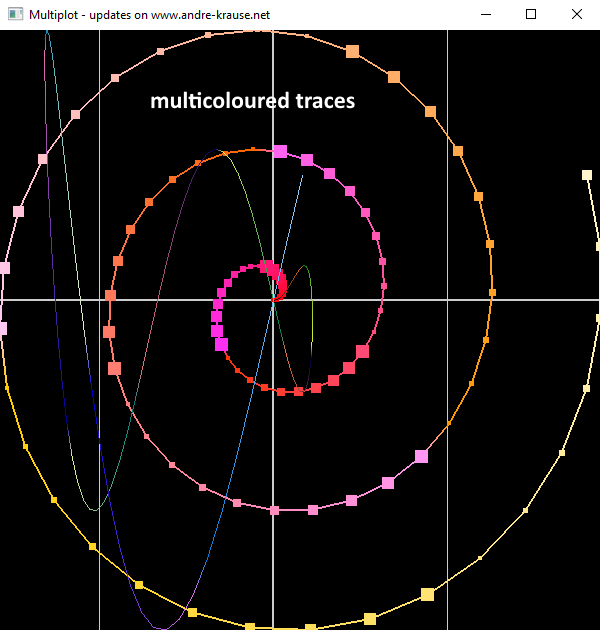# MULTIPLOT[klick for more plot examples]

With MultiPlot, you can easily plot simple graphs like line- and scatter plots in C++. It is a single - header library with zero dependencies under win32.

For other platforms, the small, lightweight and statically linkable FLTK toolkit (www.FLTK.org) is used to create an OpenGL Window. It uses www.opengl.org for plotting.

Simply include multiplot.h and start plotting. Add points with their coordinates (x,y) and optionally their colors (r,g,b) and MULTIPLOT does the rest for you. It scales automatically such that the whole graph fits to the window.

here is a minimal C++ example:

```#include "multiplot.h"
using namespace multiplot;

void main()
{
// create a multiplot Window at position (x,y)=(50,50) and width=640, height=480
Multiplot m(50, 50, 640, 480);
m.show();

// and plot a sine wave
for(int x=0; x<10000; x++)
{
m.plot(x, sin(0.1 * x) );
m.redraw();
m.check(); // event propagation
}
}
```

#### GIT

please find the latest version at: https://github.com/herculon/multiplot

#### Documentation

multiplot 0.5.6 documentation

#### History

• Version 0.5.6 26.02.2019: many fixes for linux + raspberry pi compatibility; api change: "set_" prefix removed from all member functions.
• Version 0.5.5 10.02.2019: added namespace multiplot; small bug fixes.
• Version 0.5 08.06.2016: flkt dependence removed for windows, now only win32 needed, small changes, minor modernizations, wstring for title. see multiplot.h for details
• Version 0.4 30.11.2004: minor changes and bugfixes, simplified linux build
• Version 0.3 18.10.2002: specify background - and gridcolor, line-width; create scatter-plots (plot points only)
• Version 0.2 03.10.2002: linux-configure - script, autoscaling grid display
• Version 0.1a 31.07.2002: added missing microsoft msvcr70.dll (for test_plot.exe)
• Version 0.1a 30.07.2002: removed a scaling bug, compiles now under linux
• Version 0.1 29.07.2002: Initial release

#### plot examplesPLEASE drop me a note if you have questions or something doesnt work: post@andre-krause.net
back to homepage